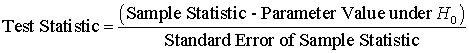### Why should I choose AnalystNotes?

Simply put: AnalystNotes offers the best value and the best product available to help you pass your exams.

##### Subject 3. Test Statistic and Significance Level
A test statistic is simply a number, calculated from a sample, whose value, relative to its probability distribution, provides a degree of statistical evidence against the null hypothesis. In many cases, the test statistic will not provide evidence sufficient to justify rejecting the null hypothesis. However, sometimes the evidence will be strong enough so that the null hypothesis is rejected and the alternative hypothesis is accepted instead.

The value of the test statistic is the focal point of assessing the validity of a hypothesis. Typically, the test statistic will be of the general form:For example, a test statistic for the mean of a distribution (such as the mean monthly return for a stock index) often follows a standard normal distribution. In such a case, the test statistic requires use of the z-test, P(Z <= test statistic = z). This is shown as:X-bar = sample mean
μ0 = hypothesized value
σ = sample standard deviation
n = sample size

Note that this assumes the population variance (and, therefore, the population standard deviation) is unknown and can only be estimated from the sample data.

There are other probability distributions as well, such as the t-distribution, the chi-square distribution, and the F-distribution. Depending on the characteristics of the population and the sample, a test statistic may follow one of these distributions, which will be discussed later.

After setting up H0 and H1, the next step is to state the level of significance, which is the probability of rejecting the null hypothesis when it is actually true. Alpha (α) is used to represent this probability. The idea behind setting the level of significance is to choose the probability that any decision will be subject to a Type I error. There is no one level of significance that is applied to all studies involving sampling. A decision by the researcher must be made to use the 0.01 level, 0.05 level, 0.10 level, or any other level between 0 and 1. A lower level of significance means that there is a lower probability that a Type I error will be made.

Learning Outcome Statements

c. explain a test statistic, Type I and Type II errors, a significance level, and how significance levels are used in hypothesis testing;

CFA® 2021 Level I Curriculum, , Volume 1, Reading 11

User Comment
maryprz14 Level of significance <<>> type I error